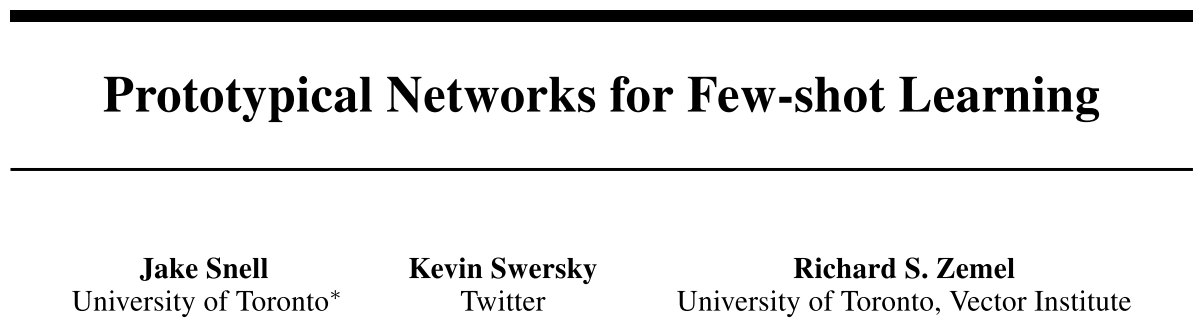## 论文信息

• 作者：Jake Snell, Kevin Swersky, Richard S. Zemel
• 出处：NIPS 2017
• 关键词：few-shot learning, metric learning
• 论文链接
• 开源代码：
• 其他资料：

## 内容简记

### 方法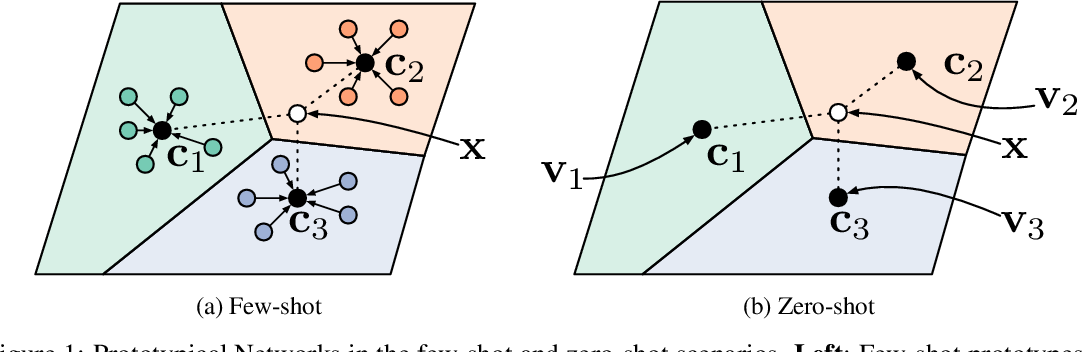### 理论分析

#### 作为混合密度估计

Prototypical Network 的学习过程可以理解为混合概率估计。Bregman 散度是一类特别的距离度量，包含欧式距离和 Mahalanobis 距离。采用 Bregman 散度时，聚类中心即是整个簇最具代表性的点（即质心），使得该类的所有点到质心的总距离之和最小。因此，Prototypical Network 使用类均值作为原型表示，并采用欧氏距离度量。而对于 Matching Network  采用的余弦距离，满足满足和簇的其他点之间总距离最小的质心是使信息损失最小化的点。

#### 作为线性模型进行解释

$$-\left|f_{\phi}(\mathbf{x})-\mathbf{c}_{k}\right|^{2}=-f_{\phi}(\mathbf{x})^{\top} f_{\phi}(\mathbf{x})+2 \mathbf{c}_{k}^{\top} f_{\phi}(\mathbf{x})-\mathbf{c}_{k}^{\top} \mathbf{c}_{k}$$

$$2 \mathbf{c}_{k}^{\top} f_{\phi}(\mathbf{x})-\mathbf{c}_{k}^{\top} \mathbf{c}_{k}=\mathbf{w}_{k}^{\top} f_{\phi}(\mathbf{x})+b_{k}, \text { where } \mathbf{w}_{k}=2 \mathbf{c}_{k} \text { and } b_{k}=-\mathbf{c}_{k}^{\top} \mathbf{c}_{k}$$

#### 与 Matching Network 的比较

Matching Network 在给定支持集的情况下生成一个加权最近邻分类器，而 Prototypical Network 使用欧氏距离生成一个线性分类器。当支持集中每个类只有一个样本时，二者等价。Matching Network 有相对复杂的结构，而 Prototypical Network 采用简单的设计达到相当甚至更好的效果。

### 实验

#### 对比实验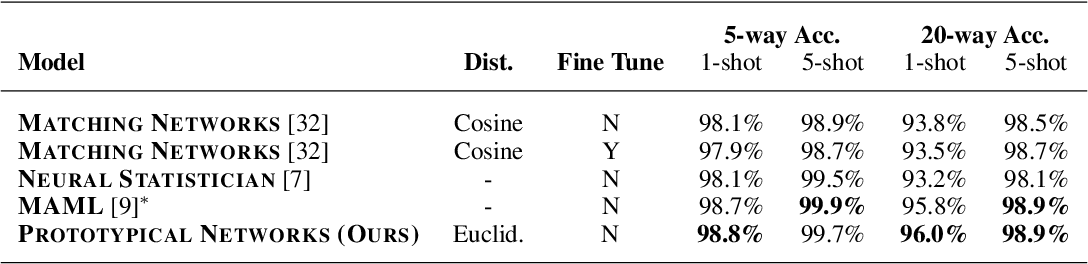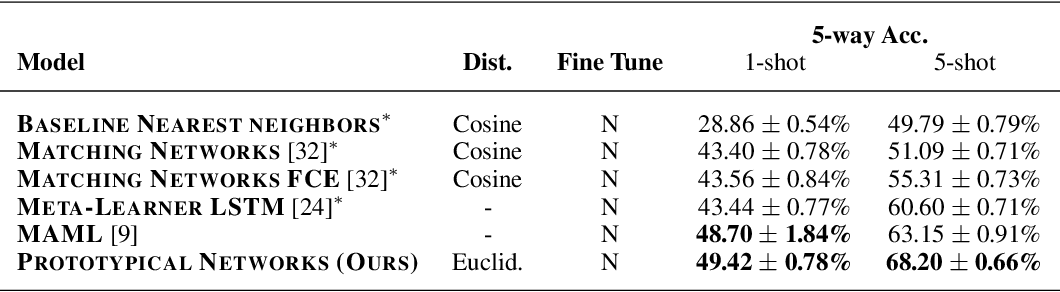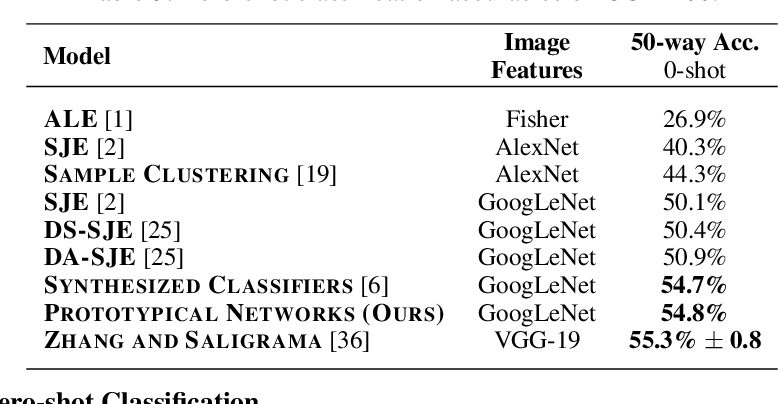1. 当训练阶段在一个任务中使用更多的类别数时，测试进行 5-way 分类的结果更好；
2. 比起 Matching Network 采用的余弦距离，欧式距离在 Prototypical Network 上的效果更好。原因已在前文进行解释。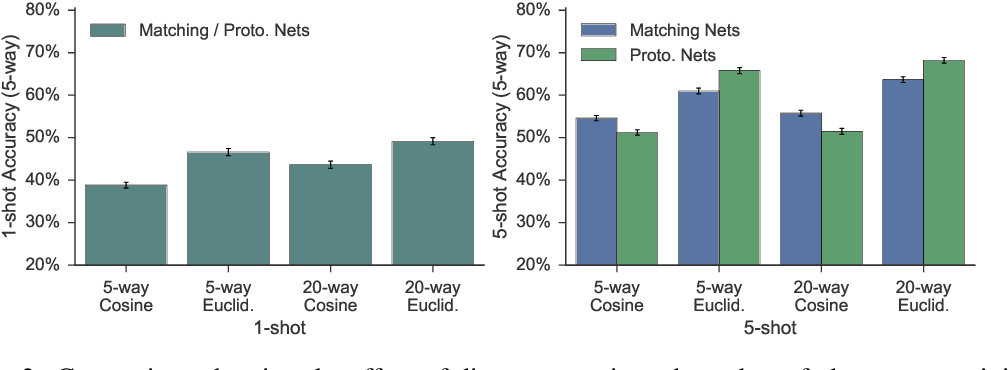## 参考论文

•  Generalizing from a Few Examples: A Survey on Few-Shot Learning, arXiv:1904.05046v2.
•  Oriol Vinyals, Charles Blundell, Tim Lillicrap, Daan Wierstra, et al. Matching networks for one shot learning. In Advances in Neural Information Processing Systems, pages 3630–3638, 2016.